GeeksforGeeks App
Open AppBrowser
Continue

# Real Life Examples of a Plane in Geometry

The word “geometry” is the English equivalent of the Greek “geometry”. “Geo” means “Earth” and “Metron” means “measure”. Even today, geometric ideas are reflected in many forms of art, measurement, textiles, design, technology, and more. For example, the shape of the ruler is different from the shape of a pencil that you write directly.

Basic Terms of Geometry with Real-Life Examples

• Plane: A plane is a two-dimensional surface with no thickness which extends infinity. It has no width. It is a flat surface. It has no boundaries. The plane has points or lines. It is a position without any thickness.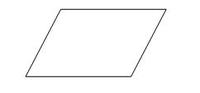Properties of a Plane

1. Two straight lines are parallel, both lines form a plane.
2. Three non-collinear points form a plane.
3. Two lines intersect forms a plane.
4. Two different planes perpendicular to a line then both planes should be parallel.

Types of Plane

1. Parallel Planes: It is defined as if 2 or more planes are parallel. Parallel planes do not intersect each other.
2. Intersecting Planes: It is defined as two or more planes are intersecting. Intersecting planes intersect in one line.

Note: In math, a plane can be formed by a line, point, or three-dimensional space. All the faces of a cuboid are planes.

### Real-Life Examples of Planes in Geometry

Planes in Geometry have many practical uses in everyday life such as measuring the circumference, area, and volume when you need to build or create something. Geometry is everywhere. Planes in Geometry allow you to determine how much material you need to complete a project. For Example – The kitchen table is a real-life example of a finite plane. Various Real-life Examples of planes in geometry are,

1. Desktop
2. Door
3. Wall
4. Whiteboard
5. A note card
6. Piece of Paper
7. A TV Screen
8. Window
9. A sheet of paper
10. A coordinate plane
11. A table
12. The part of physical space where the gravitational field is weak
13. The universe cut in half
14. The Universe
15. Outer Space
• Line: The line is a collection of points in a straight path.  It has no endpoint and no fixed length. A-Line is one-dimensional. The line has length but no width. A line is made up of a set of points that extend infinitely in opposite directions. For example, a square is made up of four lines of equal length, while a triangle is made up of three lines joined at the ends.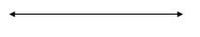Examples

1. Equator
2. The orbit of the planets.
3. Prime meridian
• Point: A point is the most important object in geometry. It is represented by a dot and it is represented by a capital letter. It has zero sizes and is represented by position only.

Examples, The tip of a needle, The intersecting point of 2 lines.

• Line Segment: It is defined as a line that has endpoints on both sides.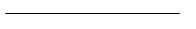Examples, Edge of the paper, pen.

• Ray: Ray is a part of the line. It has one endpoint and no fixed length. A ray can be extended infinitely in any one direction. Sunlight is an example of a ray. A ray is also called a half-line. We do not measure the length of a ray.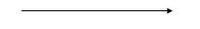Examples, Sun Rays, Flashlight, Arrows.

• Intersecting Line: It is defined as when 2 lines share the same point.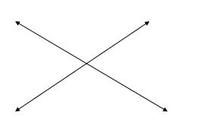Examples, The hands of the clock.

• Perpendicular Line: It is defined as when 2 lines meet at a point and form a right angle among themselves.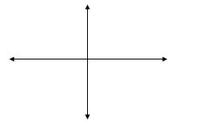Examples, Door edges, The equator, and prime meridian.

• Parallel Line: It is defined as when 2 lines are parallel to each other if they never intersect each other.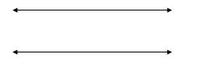Examples, Railway tracks, Lines on a road.

### Conceptual Questions

Question 1: Why is a plane two-dimensional?

The plane is two-dimensional because the length of a rectangle is independent of its width. Every point in a plane can be described by a linear combination of 2 independent vectors.

Question 2: How do you represent a plane in geometry?

Plane in geometry is represented in drawings by the four-sided figure. A single capital letter is used to denote a plane. A plane has infinite length, infinite width, and zero thickness.

Question 3: Give a real-life example of a finite plane. Is it possible to have a real-life object in an infinite plane?

A Real-life Example of the finite plane is the Kitchen table. No, It is not possible to have real objects in an infinite plane because all real objects have edges which means they are finite.

Question 4: What is a plane in real life?

A plane is a flat surface with no thickness. The world has 3 dimensions but there are only 2 dimensions on a plane that is length and width makes a plane.

Question 5: Does the plane extend forever?

Yes, the Plane extends forever in two dimensions but they have no thickness. A plane is a flat surface that contains infinitely many intersecting lines that extend forever in all directions.

Question 6: How do you define a plane in 3d?